# A Survey of Zero-Shot Learning

## Settings, Methods, and Applications

Posted by JoselynZhao on April 15, 2019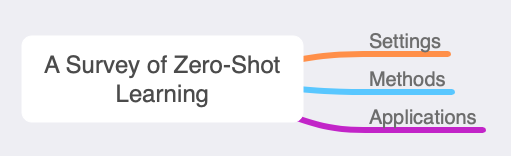Zero-shot learning is a powerful and promising learning paradigm, in which the classes covered by training instances and the classes we aim to classify are disjoint.

In this paper

1. provide an overview of zero-shot learning. classify zero-shot learning into three learning settings.
2. describe different semantic spaces adopted in existing zero-shot learning works.
3. categorize existing zero-shot learning methods and introduce representative methods under each category.
4. discuss different applications of zero-shot learning
5. highlight promising future research directions of zero-shot learning

## 1 Introduction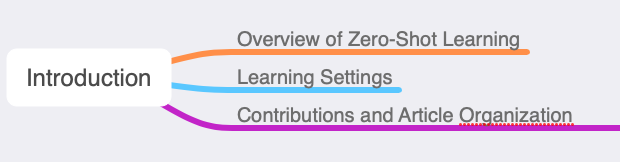#### restrictions(限制)

In supervised classification:

• need sufficient labels.
• the learned classifier can only classify the instances belonging to classes covered by the training data

#### Existing plan

open set recognition methodsLalit P. Jain, Walter J. Scheirer, and Terrance E. Boult. 2014. Multi-class open set recognition using probability of inclusion. In European Conference on Computer Vision (ECCV’14). 393–409.

it cannot determine which specific unseen class the instance belongs to.

For methods under the above learning paradigms, if the testing instances belong to unseen classes that have no available labeled instances during model learning (or adaption), the learned classifier cannot determine the class labels of them.

which require the classifier to have the ability to determine the class labels for the instances.

• The number of target classes is large collecting sufficient labeled instances for such a large number of classes is challenging.
• Target classes are rare. An example is fine-grained object classification. For many rare breeds, we cannot find the corresponding labeled instances.

• Target classes change over time. for some new products, it is difficult to find corresponding labeled instances
• In some particular tasks, it is expensive to obtain labeled instances. For example, in the image semantic segmentation problem

To solve this problem, zero-shot learning (also known as zero-data learning ) is pro- posed.

The aim of zero-shot learning: classify instances belonging to the classes that have no labeled instances.

range of applications:

• computer vision
• natural language processing
• ubiquitous computing

### 1.1 Overview of Zero-Shot Learning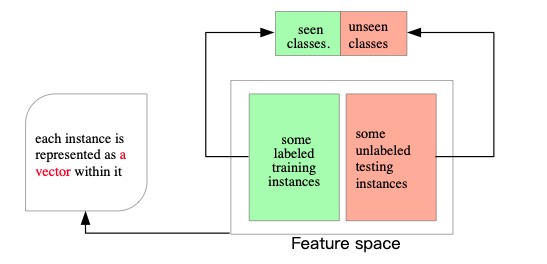Each instance is usually assumed to belong to one class.

#### the definition of zero-shot learning

Denote $S=\left { c_{i}^{s} i = 1,2,…,N_{s}\right }$ as the set of seen classes Denote $U=\left { c_{i}^{u} i = 1,2,…,N_{u}\right }$ as the set of unseen classes Note that S∩U = ∅.

Denote X as the feature space, which is D dimensional Denote $D^{tr} = \left { (x_{i}^{tr},y_i^{tr}) \in X \times S \right }{i=1}^{N{tr}}$ as the set of labeled training instances belonging to seen classes;

Denote $X^{te} = \left{x_i^{te} \in X \right} {i=1}^{N{te}}$ as the set of testing instances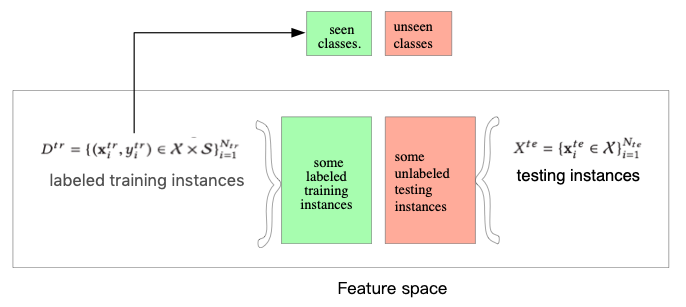Definition 1.1 (Zero-Shot Learning). Given labeled training instances $D^{tr}$ belonging to the seen classes S, zero-shot learning aims to learn a classifier$f^u(·)$ : X→U that can classify testing instances$X^{te}$ (i.e., to predict $Y^{te}$ ) belonging to the unseen classes U.

zero-shot learning is a subfield of transfer learning（迁移学习）

#### transfer learning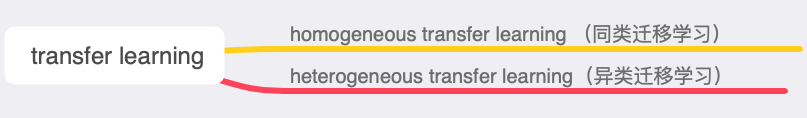In homogeneous transfer learning： the feature spaces and the label spaces are the same in heterogeneous transfer learning： the feature spaces and/or the label spaces are different.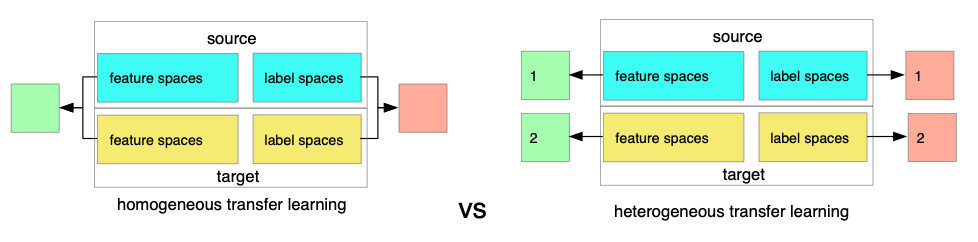In zero-shot learning： the same feature spaces, but different label spaces.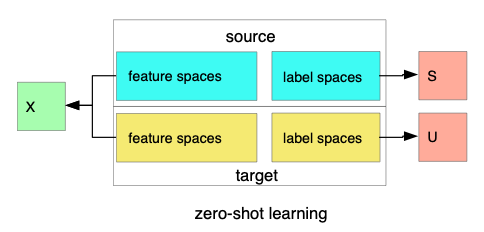so zero-shot learning belongs to heterogeneous transfer learning.

note: heterogeneous transfer learning with different label spaces(HTL-DLS)

HTL-DLS VS zero-shot learning: whether there are some labeled instances for the target label space classes. HTL-DLS have, however zero-shot learning dose not.

#### Auxiliary information(辅助信息)

Such auxiliary information should contain information about all of the unseen classes. Meanwhile, the auxiliary information should be related to the instances in the feature space. the auxiliary information involved by existing zero-shot learning methods is usually some semantic information. It forms a space that contains both the seen and the unseen classes.

We denote $\tau$ as the semantic space. Suppose $\tau$ is M-dimensional. Denote $t_i^s \in \tau$ as the class prototype for seen class $c_i^s$. Denote $t_i^u \in \tau$ as the class prototype for unseen class $c_i^u$.

Denote $T^s = \left{t_i^s \right}{i=1}^{N_s}$ as the set of prototypes for seen classes Denote $T^u = \left{t_i^u \right}{i=1}^{N_u}$ as the set of prototypes for unseen classes

Denote π (·) : S∪U→T as a class prototyping function that takes a class label as input and outputs the corresponding class prototype.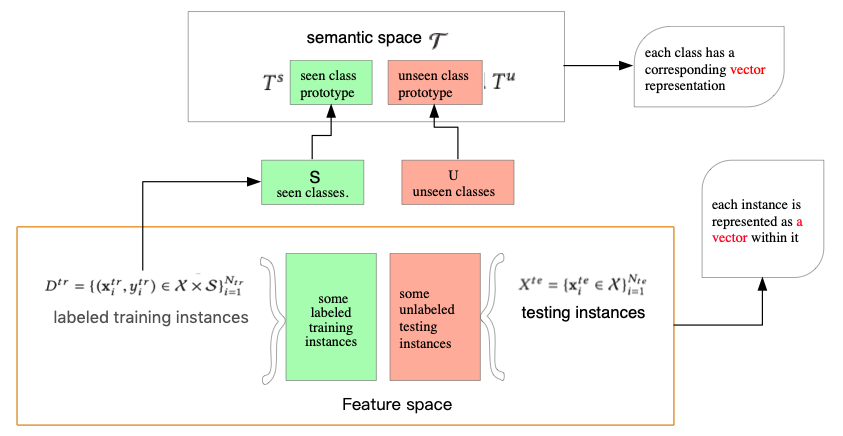We summarise the key notations used throughout this article in Table 1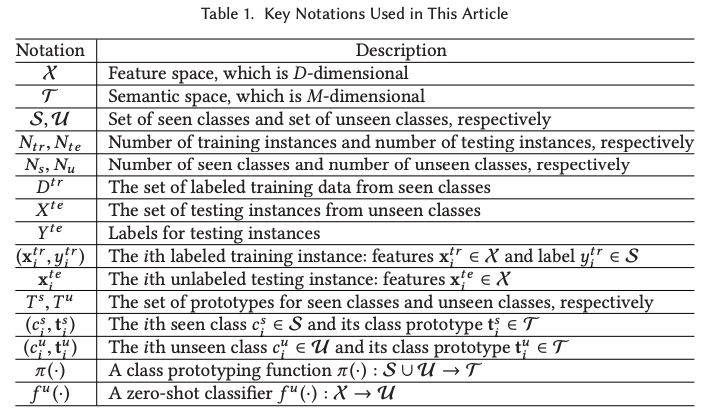### 1.2 Learning Settings

Based on the degree of transduction, we categorise zero-shot learning into three learning settings.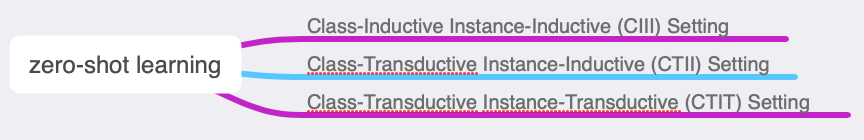Definition 1.2 (Class-Inductive Instance-Inductive (CIII) Setting). Only labeled training instances $D^{tr}$ and seen class prototypes $T^s$ are used in model learning.

Definition 1.3 (Class-Transductive Instance-Inductive (CTII) Setting). Labeled training instances $D^{tr}$ , seen class prototypes $T^s$ , and unseen class prototypes $T^u$ are used in model learning.

Definition 1.4 (Class-Transductive Instance-Transductive (CTIT) Setting). Labeled training instances$D^{tr}$ , seen class prototypes$T^s$, unlabeled testing instances $X^{te}$ , and unseen class prototypes $T^u$ are used in model learning.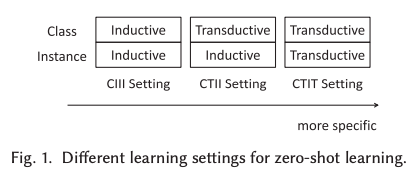from the fig.1. we can see the classifier $f^u (·)$ is learned with increasingly specific testing instances’ information.

the performance of the model learned with the training instances will decrease when applied to the testing instances. In zero-shot learning, this phenomenon is usually referred to as domain shift （Yanwei Fu, Timothy M. Hospedales, Tao Xiang, and Shaogang Gong. 2015. Transductive multi-view zero-shot learning. IEEE Transactions on Pattern Analysis and Machine Intelligence 37, 11 (2015), 2332–2345.）

### 1.3 Contributions and Article Organization

Based on how the feature space and the semantic space are related, this article categorises the zero-shot learning methods into three categories：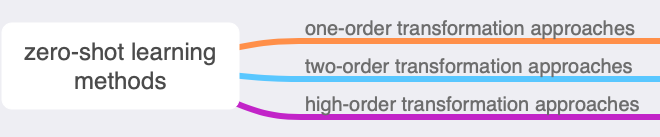in this article, the emphasis is the evaluation of existing zero-shot learning methods.

a comprehensive survey of zero-shot learning that covers a systematic categorisation of learning settings, methods, semantic spaces, and applications is needed.

#### Our contributions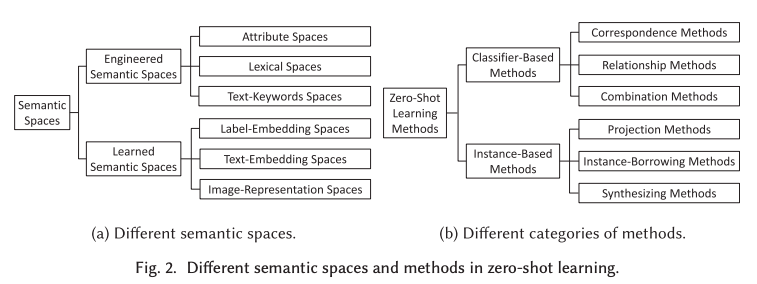1. As shown in Figure 2(b), we provide a hierarchical categorisation of existing methods in zero-shot learning.
2. We provide a formal classification and definition of different learning settings in zero-shot learning.
3. As shown in Figure 2(a), we provide a categorisation of existing semantic spaces in zero-shot learning.

#### Article organization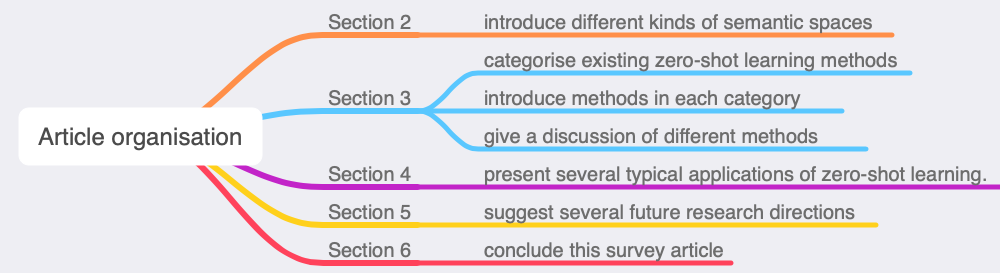## 2 SEMANTIC SPACES

According to how a semantic space is constructed，the semantic space can be divided as follows：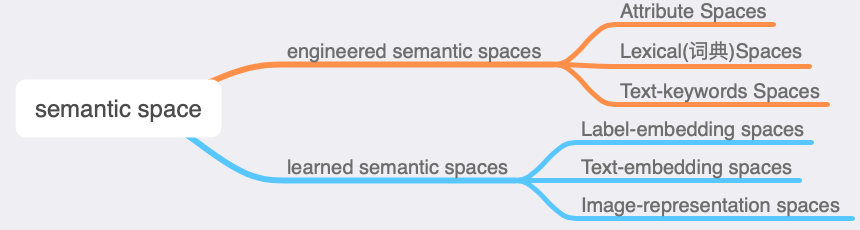### 2.1 Engineered Semantic Spaces

#### Attribute spaces

Attribute spaces are constructed by a set of attributes. In an attribute space, a list of terms describing various properties of the classes are defined as attributes.

Each attribute is usually a word or a phrase corresponding to one property（性能） of these classes.

Then, these attributes are used to form the semantic space. with each dimension being one attribute.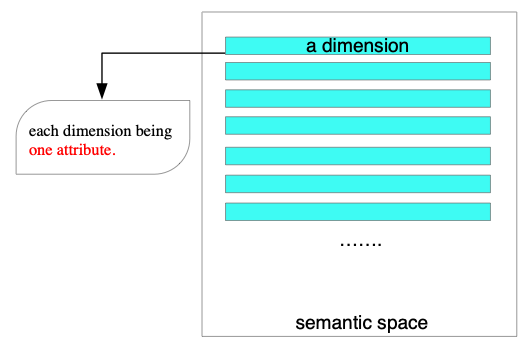For each class, the values of each dimension of the corresponding prototype are determined by whether this class has a corresponding attribute.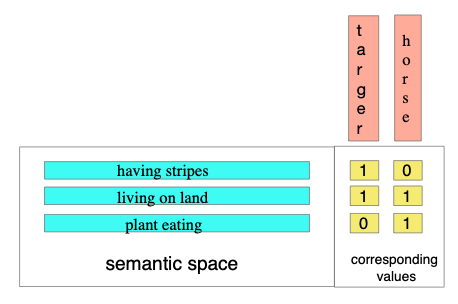so the attribute values are binary (i.e., 0/1). the resulting attribute space is referred to as a binary attribute space.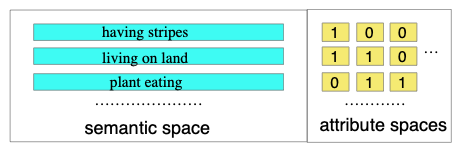there also exist relative attribute spaces, which measure the relative degree of having an attribute among different classes. (Devi Parikh and Kristen Grauman. 2011. Relative attributes. In Proceedings of the IEEE International Conference on Computer Vision (ICCV’11). 503–510)

#### Lexical spaces

Lexical spaces are constructed by a set of lexical items(词汇项)

Lexical spaces are based on the labels of the classes and datasets that can provide semantic information.

#### Text-keyword spaces

Text-keyword spaces are constructed by a set of keywords extracted from the text descriptions of each class.

both the Plant Database and Plant Encyclopedia are used (which are specific for plants) to obtain the text descriptions for each flower class.

In zero-shot video event detection, the text descriptions of the events can be obtained from the event kits provided in the dataset.

After obtaining the text descriptions for each class, the next step is to construct the semantic space and generate class prototypes from these descriptions.

each dimension corresponding to a keyword.

#### Summary of engineered semantic spaces

The advantage of engineered semantic spaces： the flexibility to encode human domain knowledge through the construction of semantic space and class prototypes.

The disadvantage of engineered semantic spaces： the heavy reliance on humans to perform the semantic space and class prototype engineering

### 2.2 Learned Semantic Spaces

the semantic information is contained in the whole prototype.

#### Label-embedding spaces

the class prototypes are obtained through the embedding of class labels.

In word embedding: words or phrases are embedded into a real number space as vectors. In this space, semantically similar words or phrases are embedded as nearby vectors

In zero-shot learning, for each class, the class label of it is a word or a phrase.

In addition to generating one prototype for each class, there are also works [103, 125] that generate more than one prototype for each class in the label embedding space. In these works, the prototypes of a class are usually multiple vectors following Gaussian distribution（高斯分布）.

#### Text-embedding spaces

the class prototypes are obtained by embedding the text descriptions for each class.（Being similar to text-keyword spaces）

the major difference between above two： text-keyword space is constructed through extracting keywords and using each of them as a dimension in the constructed space. A text-embedding space is constructed through some learning models.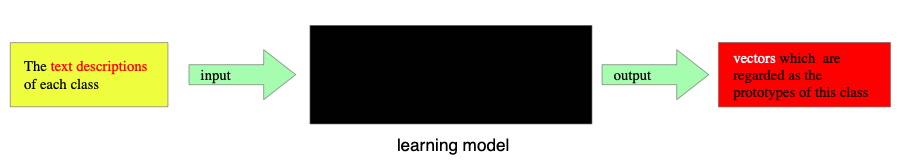#### Image-representation spaces

the class prototypes are obtained from images belonging to each class. (类似 text-embedding spaces)

#### Summary of learned semantic spaces

The advantage： the process of generating them is relatively less labor intensive, and the generated semantic spaces contain information that can be easily overlooked by humans.

The disadvantage: the prototypes of classes are obtained from some machine-learning models, and the semantics of each dimension are implicit.

## 3 METHODS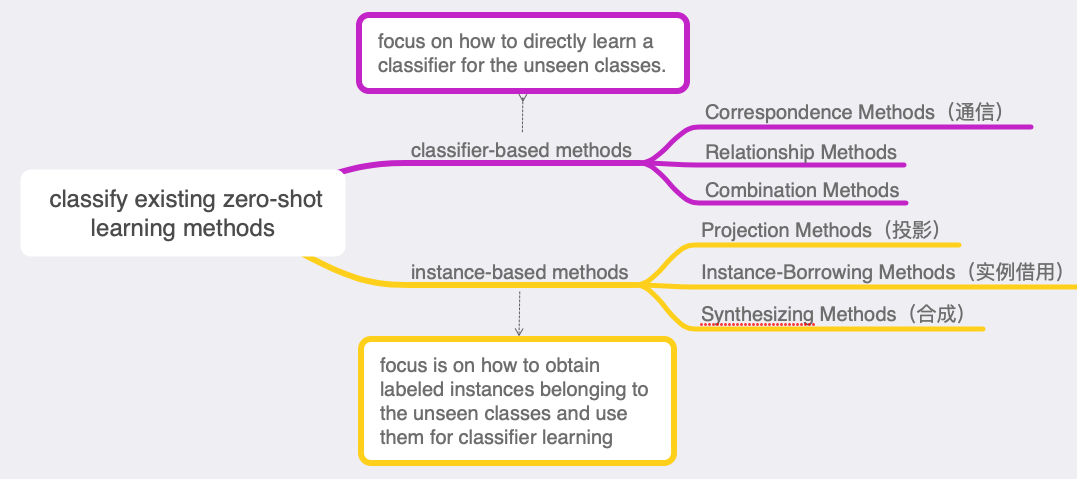for a zero-shot learning task, we consider one semantic space and represent each class with one prototype in that space.

### 3.1 Classifier-Based Methods

Existing classifier-based methods usually take a one-versus-rest（一对多） solution for learning the multiclass zero-shot classifier $f^u(·)$.

denote $f_i^u(·)： R^D →$ {0, 1} as the binary one-versus-rest classifier for class $c_i^u \in U$. the eventual zero-shot classifier$f^u$ (·) for the unseen classes consists of $N_u$ binary one-versus-rest classifiers { $f_i^u (·) | i=1,2,…,N_u$ }.

#### 3.1.1 Correspondence Methods